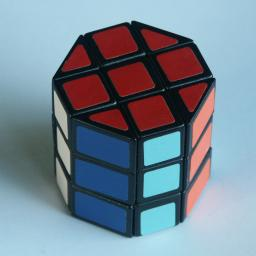# Barrel/OctagonThis variation on the Rubik's cube is called the Magic Octagonal Prism, or just The Barrel or The Octagon. In Dutch it is called 'Peperbus', which means pepperpot. It is exactly the same as the normal cube except that the four vertical edges of the cube are truncated. The corner pieces of original cube now have only two colours and the four edge pieces in the middle layer have only one colour.

The differences make this puzzle a little confusing. It is possible to get to a position where it seems that two pieces need to be swapped or where one edge piece needs to be flipped, both of which are impossible on the original cube.

## The number of positions:

There are 8 corner pieces with 3 orientations each, 12 edge pieces with 2 orientations each, giving a maximum of 8!·12!·38·212 positions. This limit is not reached because:
• The total twist of the corners is fixed (3)
• The middle layer edge pieces show no orientation (24)
• The colours of the vertical columns are equivalent (4!)

This leaves 8!·12!·37·28/4! = 450,541,700,775,936,000 or 4.5·1017 positions.

## Solution:

Use any solution method for the Rubik's Cube to solve it as far as possible. As mentioned above, there are two situations that may occur that cannot happen on a normal cube.

• One edge needs to be flipped: Simply flip that edge and one of the shaved (monochrome) edges. One neat method (which actually flips 3 shaved edges) is to hold the barrel with the flipped edge at the UR location, where U is an octagon face, and do E R E R E R E R, where E denotes turning the horizontal middle slice to the right (anti-clockwise looking from above).
• Two pieces need to be swapped To swap two pieces just swap any two of the shaved columns. For example if the U face is an octagonal face, then RL U2 R'L' will swap the UF and UB edges. Alternatively R2 F2 R2 swaps the UF and DF edges.

Some people prefer to hold the puzzle so that the last layer they solve is one with two shaved edges (e.g. the octagonal sides are at L and R). This way any final flips/swaps only involve pieces of the final layer.